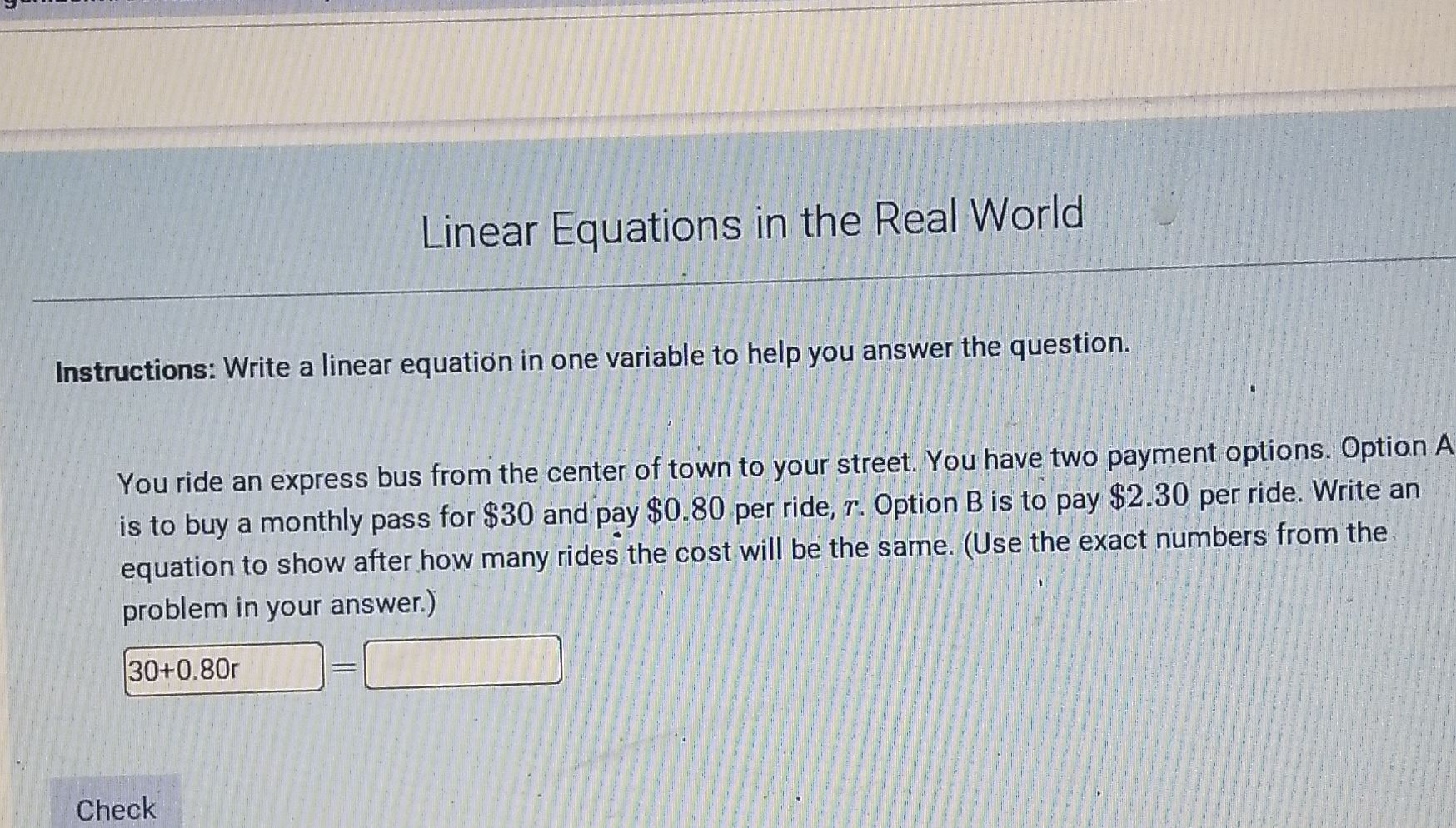### Still have math questions?You ride an express bus from the center of town to your street. You have two payment options. Option A is to buy a monthly pass for $$\ 30$$ and pay $$\ 0.80$$ per ride, $$r$$ . Option B is to pay $$\ 2.30$$ per ride. Write an equation to show after how many rides the cost will be the same. (Use the exact numbers from the. problem in your answer.)